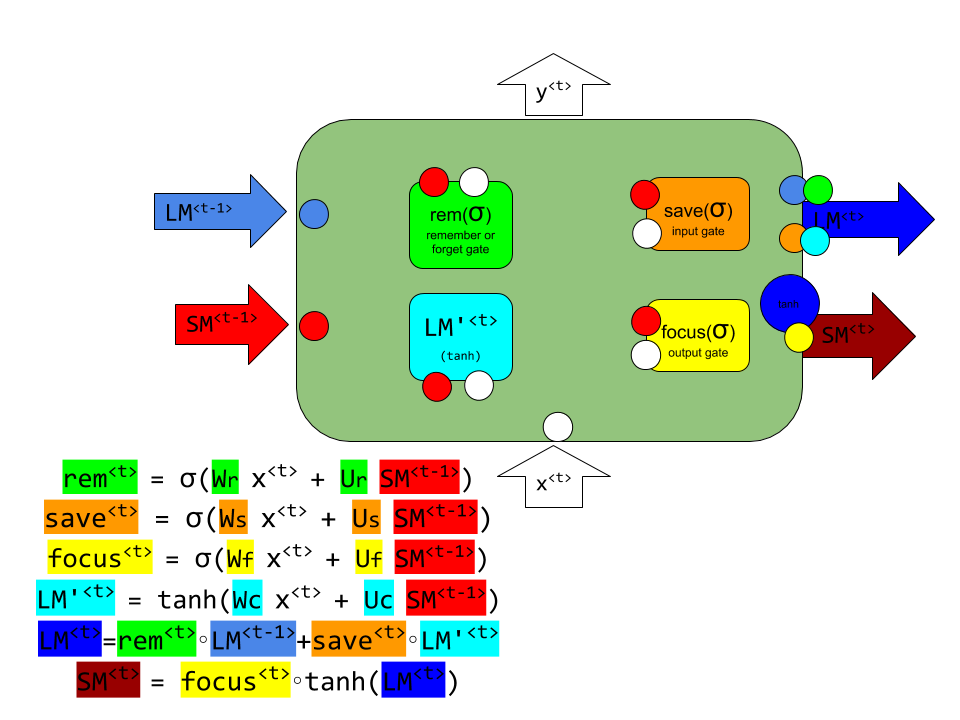Here is the model of LSTM cell with the following notation:

• LM ( long memory, same as c = captivated state )
• SM ( short memory, same as h = hidden state )
• Remember gate ( same as forget gate )
• Save gate ( or input gate)
• Focus gate ( or output gate )
• LM’ ( new state, that may or may not replace the LM state )In PyTorch you can:

• implement the LSTM from scratch,
• use torch.nn.LSTM class
• use torch.nn.LSTMCell class

### LSTM Net

Having many LSTM cells we form LSTM layers. Many LSTM layers we stack into LSTM networks. The next image presents the LSTM network with two LSTM layers and in between the dropout layer.

_ _ _ _ _ ... _
. . . . . ... .
_ _ _ _ _ ... _


We would create this with the following code

nn.LSTM(    input_size=128,        # input features
hidden_size=128,       # hidden units (features)
num_layers=2,          # number of stacked layres
dropout=0.2 )          # only if num_layers > 1


In here num_layers=2, default is: 1. We call LSTM networks stacked LSTM when two or more LSMT layers.

dropout parameter of torch.nn.LSTM constructor by default is 0. If non-zero, introduces a Dropout layer on the outputs of each LSTM layer except the last layer.

input_size parameter of torch.nn.LSTM constructor defines the number of expected features in the input x.

hidden_size parameter of torhc.nn.LSTM constuctor defines the number of features in the hidden state h. hidden_size in PyTorch equals the numer of LSTM cells in a LSMT layer.

In total there are hidden_size * num_layers LSTM cells (blocks) like in the image we above.